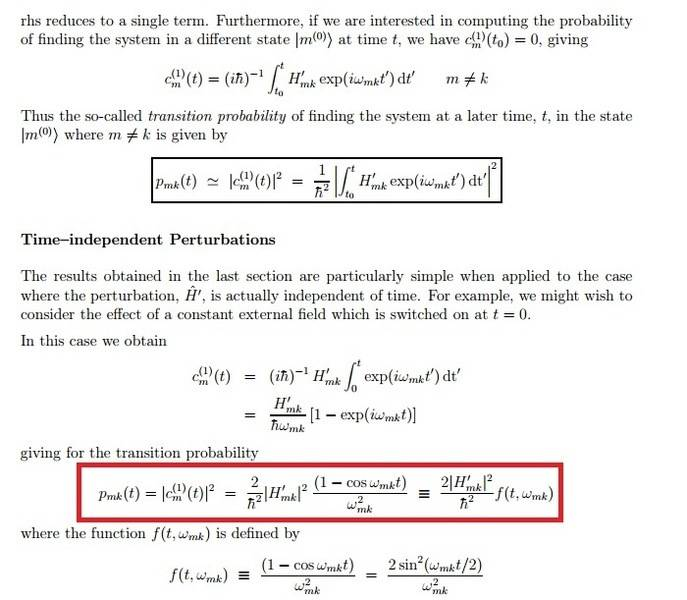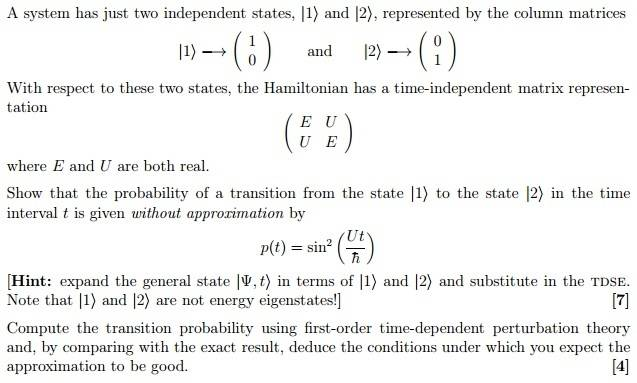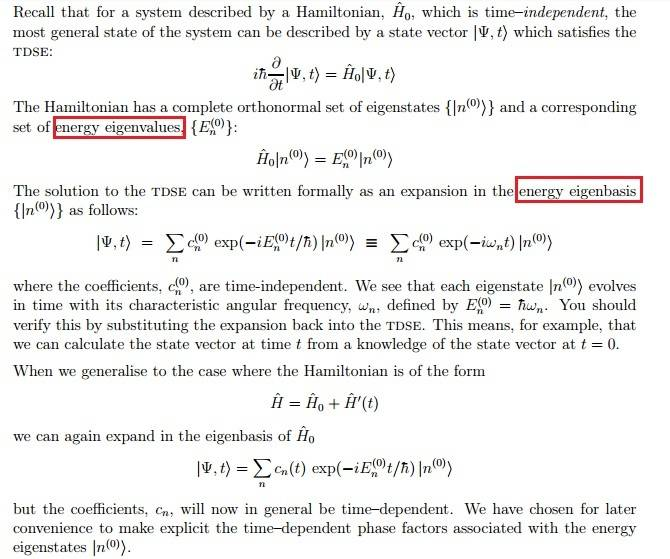# Time-dependent perturbation theory

• davon806

## Homework Statement

The problem consists of 2 parts,the first one(I have done it) is on the following website:

Q1: I calculated the desired result p(t) = sin^2(Ut/h). However,I don't understand why <1,t | 2 > will give the coefficient corresponds to the transition probability from state 1 to state 2 in Time t. My initial guess is similar to that of OP in the above post, but take the scalar product of the general state with |2> .

In the second part,I was asked to compute the transition probability using 1st-order time-dependent perturbation theory.Using the result from my notes:where P_mk means the transition probability from energy eigenstate k to m at time t.

H'_mk = < m(0) | H' | k(0) > , where H' denotes the perturbation.

w_mk = w_m - w_k = ( E_m - E_k )/ h

Q2: I was a bit confused when I calculate H'_mk. Using the definition of scalar product in matrix
representation, I have H'_21 = < u_2 | H' | u_1> , where |u_i> is defined as in the above post.
However,(1 -1)(E U U E) (1 1)(forgive me for the matrix notation...) is 0 since |u_1> and |u_2>
are orthogonal. So the P_21 (t) vanishes which is not the thing I want.

Interestingly, if instead, I take the state m and k to be |2> and |1> respectively, I would have
(0 1)(E U U E) (1 0) = U ,and following the formula I found that P_21 (t) = sin^2(Ut/h)

However,(0 1) and (1 0) are NOT energy eigenstates,but H'_mk works with energy eigenstate.Why would they produce the desired result?

2. Homework Equations

Note that I have calculated that E_1 = E+U and E_2 = E-U where E_1 is the (energy) eigenvalue of |u_1> and etc.

For a clearer version of the original question:## The Attempt at a Solution

Incorporated in question

Last edited:

Q1: I calculated the desired result p(t) = sin^2(Ut/h). However,I don't understand why <1,t | 2 > will give the coefficient corresponds to the transition probability from state 1 to state 2 in Time t.
This is basically Born's rule. The probability of finding the system described by ##| \psi \rangle## in state ##| \phi \rangle## is given by ##\left| \langle \phi | \psi \rangle \right|^2##. Since the system is initially in state ##| 1 \rangle##, i.e., ##| \psi (t=0) \rangle = | 1 \rangle##, calculating ##\left| \langle 2 | \psi (t) \rangle \right|^2## will give the transition probability from ##| 1 \rangle## to ##| 2 \rangle## as a function of time.

Q2: I was a bit confused when I calculate H'_mk. Using the definition of scalar product in matrix
representation, I have H'_21 = < u_2 | H' | u_1> , where |u_i> is defined as in the above post.
However,(1 -1)(E U U E) (1 1)(forgive me for the matrix notation...) is 0 since |u_1> and |u_2>
are orthogonal. So the P_21 (t) vanishes which is not the thing I want.

Interestingly, if instead, I take the state m and k to be |2> and |1> respectively, I would have
(0 1)(E U U E) (1 0) = U ,and following the formula I found that P_21 (t) = sin^2(Ut/h)

However,(0 1) and (1 0) are NOT energy eigenstates,but H'_mk works with energy eigenstate.Why would they produce the desired result?

In the former case, you simply showed that eigenstates of a time-independent Hamiltonian are constants of motion. It is the second approach that you want here.
Once you decide on a basis set representation, you stick to it! If the system is expressed in the ##\left\{ | 1 \rangle, | 2 \rangle \right\}## basis, then the matrix elements of the Hamiltonian are calculated in that basis, not the eigenstate basis. You can of course decide to work in the eigenstate basis, but then you have to change the vector representation of ##| 1 \rangle## and ##| 2 \rangle##.

This is basically Born's rule. The probability of finding the system described by ##| \psi \rangle## in state ##| \phi \rangle## is given by ##\left| \langle \phi | \psi \rangle \right|^2##. Since the system is initially in state ##| 1 \rangle##, i.e., ##| \psi (t=0) \rangle = | 1 \rangle##, calculating ##\left| \langle 2 | \psi (t) \rangle \right|^2## will give the transition probability from ##| 1 \rangle## to ##| 2 \rangle## as a function of time.

In the former case, you simply showed that eigenstates of a time-independent Hamiltonian are constants of motion. It is the second approach that you want here.
Once you decide on a basis set representation, you stick to it! If the system is expressed in the ##\left\{ | 1 \rangle, | 2 \rangle \right\}## basis, then the matrix elements of the Hamiltonian are calculated in that basis, not the eigenstate basis. You can of course decide to work in the eigenstate basis, but then you have to change the vector representation of ##| 1 \rangle## and ##| 2 \rangle##.

Thanks! I was really happy that someone replies my lengthy and messy post! I understand Q1 but not quite for Q2:

1."In the former case, you simply showed that eigenstates of a time-independent Hamiltonian are constants of motion." ... Why did this imply
the eigenstates of H' is a constant of motion?

2.Below is the first page of my notes(the derivation of time-dependent perturbation)Notice the red boxes,everything used in the derivation is the energy eigenstates.I don't understand why |1> and |2> are related to the context.
|1> and |2> actually represents the spin-up and spin-down ,so I think there is nothing to do with energy..?

1."In the former case, you simply showed that eigenstates of a time-independent Hamiltonian are constants of motion." ... Why did this imply
the eigenstates of H' is a constant of motion?
What you showed is that if the system is in state ##|u_1 \rangle## or ##|u_2 \rangle##, which are eigenstates of the Hamiltonian, then the system will stay forever in those states, which is what you would expect for a time-independent Hamiltonian.

2.Below is the first page of my notes(the derivation of time-dependent perturbation)
Notice the red boxes,everything used in the derivation is the energy eigenstates.I don't understand why |1> and |2> are related to the context.
|1> and |2> actually represents the spin-up and spin-down ,so I think there is nothing to do with energy..?
This is all fine in the abstract Dirac notation. But when you go to matrix-vector notation, you have to make a choice of basis. In the problem, it is stated the basis used is ##|1\rangle \rightarrow (1, 0)^T## and ##|2\rangle \rightarrow (0, 1)^T ##. It is in that basis that
$$\hat{H} \rightarrow \begin{pmatrix} E & U \\ U & E \end{pmatrix}$$
such that the matrix elements ##H_{1,2} = H_{2,1} = U##.

Note that in terms of the notes you have posted, ##|1 \rangle## and ##|2 \rangle## are eigenstates of ##\hat{H}_0##,
$$\hat{H}_0 \rightarrow \begin{pmatrix} E & 0 \\ 0 & E \end{pmatrix}$$
and the coupling is given by
$$\hat{H}' \rightarrow \begin{pmatrix} 0 & U \\ U & 0 \end{pmatrix}$$
So you are working with eigenstates of the base Hamiltonian ##\hat{H}_0##.

This is all fine in the abstract Dirac notation. But when you go to matrix-vector notation, you have to make a choice of basis. In the problem, it is stated the basis used is ##|1\rangle \rightarrow (1, 0)^T## and ##|2\rangle \rightarrow (0, 1)^T ##. It is in that basis that
$$\hat{H} \rightarrow \begin{pmatrix} E & U \\ U & E \end{pmatrix}$$
such that the matrix elements ##H_{1,2} = H_{2,1} = U##.

Note that in terms of the notes you have posted, ##|1 \rangle## and ##|2 \rangle## are eigenstates of ##\hat{H}_0##,
$$\hat{H}_0 \rightarrow \begin{pmatrix} E & 0 \\ 0 & E \end{pmatrix}$$
and the coupling is given by
$$\hat{H}' \rightarrow \begin{pmatrix} 0 & U \\ U & 0 \end{pmatrix}$$
So you are working with eigenstates of the base Hamiltonian ##\hat{H}_0##.

Thanks for clarifying the notations and it makes much more sense to me

My last question: Can you explain why can we decompose the matrix as H_0 and H' as you have shown?
And if H' = ( 0 U U 0) instead of ( E U U E) , then H'_21 = (0 1) (0 U U 0) (1 0) = (U) ?

My last question: Can you explain why can we decompose the matrix as H_0 and H' as you have shown?
You have that ##\hat{H} = \hat{H}_0 + \hat{H}'##, so you can decompose it any way you want, so long as both ##\hat{H}_0## and ##\hat{H}'## are hermitian. The most natural approach is to take ##\hat{H}_0## to be the diagonal, and ##\hat{H}'## to something that only induces couplings between the eigenstates of ##\hat{H}_0##. (Most often, ##\hat{H}_0## describes the base system and ##\hat{H}'## an external factor).

And if H' = ( 0 U U 0) instead of ( E U U E) , then H'_21 = (0 1) (0 U U 0) (1 0) = (U) ?
Yes. But note that you are mixing two approaches. Given the basis set ##\left\{ | \phi_i \rangle \right\}##, the state of the system in vector notation is given by
$$| \psi \rangle \rightarrow \begin{pmatrix} \langle \phi_1 | \psi \rangle \\ \langle \phi_2 | \psi \rangle \\ \vdots \end{pmatrix}$$
and any operator ##\hat{A}## is described by a matrix ##\mathbf{A}##, with elements obtained as ##A_{i,j} = \langle \phi_i | \hat{A} | \phi_j \rangle##.

If you are given matrix ##\mathbf{A}##, then you can simply find the elements ##A_{i,j}## by reading them off the matrix.

•davon806